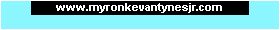Program1031.comTMGame Programming Help for Beginners! Home Need Help? Start here!Questions and Answers Get Exclusive Stuff!Email Newsletter

Question:
How do I Make a Positive Number Negative in Game Maker?

Want to make a positive number negative? Here's how!

Let's say we want to make a variable negative. Our variable is called myDirection and it equals 5:

 myDirection=5;

How do we make this negative? Remember, any number multiplied by 1 gives you the same number, and multiplying by any negative number gives you a negative number answer. So with that in mind, to make it negative, just multiply it by a negative 1. So just insert this code:

 myDirection=myDirection*-1;

This code multiplies the value of myDirection by -1, and then puts the answer back in the myDirection variable. Now myDirection is negative! Sweet! Try it in your own projects!

 Page updated: March 23rd, 2015 @ 6:21 AM Eastern Time

 Our other websites: